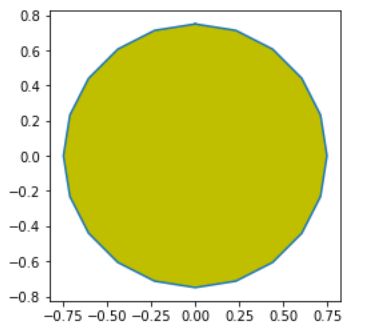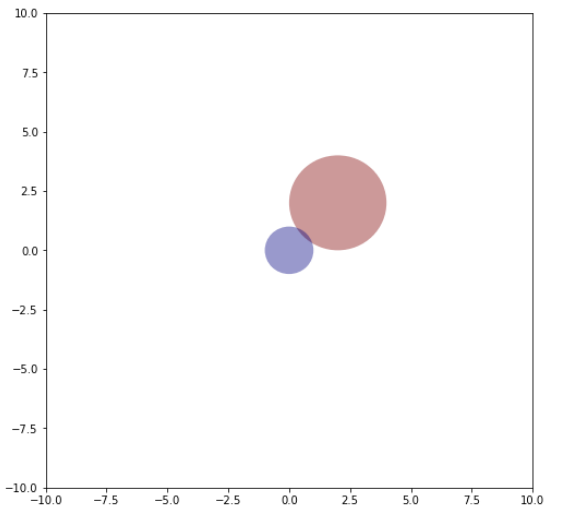# Matplotlib.patches.CirclePolygon class in Python

Matplotlib is an amazing visualization library in Python for 2D plots of arrays. Matplotlib is a multi-platform data visualization library built on NumPy arrays and designed to work with the broader SciPy stack.

## matplotlib.patches.CirclePolygon

The `matplotlib.patches.CirclePolygon` class is used for polygon-approximation of a circle patch. It is used to make a circle at `xy = (x, y)` with a radius that has been provided. A regular polygon with resolution sides is approximated with this circle.

Syntax: class matplotlib.patches.CirclePolygon(xy, radius=5, resolution=20, **kwargs)

Parameters:

• xy: The origin coordinate of the circle to be drawn.
• radius: It’s an optional parameter that is used to set the radius of the circle.It defaults to 5 units.
• resolution: As the name suggest it is used to set the image resolution. It is optional and defaults to 20.

Below table provides with the optional valid kwargs ;

PROPERTY DESCRIPTION
agg_filter a filter function that takes a (m, n, 3) float array and a dpi value that returns a (m, n, 3) array
alpha float or None
animated bool
antialiased or aa unknown
capstyle {‘butt’, ’round’, ‘projecting’}
clip_box Bbox
clip_on bool
clip_path [(Path, Transform)|Patch|None]
color color or sequence of rgba tuples
contains callable
edgecolor or ec or edgecolors color or None or ‘auto’
facecolor or fc or facecolors color or None
figure figure
fill bool
gid str
hatch {‘/’, ‘\’, ‘|’, ‘-‘, ‘+’, ‘x’, ‘o’, ‘O’, ‘.’, ‘*’}
in_layout bool
joinstyle {‘miter’, ’round’, ‘bevel’}
linestyle or ls {‘-‘, ‘–‘, ‘-.’, ‘:’, ”, (offset, on-off-seq), …}
linewidth or linewidths or lw float or None
path_effects AbstractPathEffect
picker None or bool or float or callable
path_effects AbstractPathEffect
picker float or callable[[Artist, Event], Tuple[bool, dict]]
rasterized bool or None
sketch_params (scale: float, length: float, randomness: float)
snap bool or None
transform matplotlib.transforms.Transform
url str
visible bool
zorder float

Example 1:

 `import` `matplotlib.pyplot as plt ` `from` `matplotlib.patches ``import` `CirclePolygon ` ` `  ` `  `circle ``=` `CirclePolygon((``0``, ``0``),  ` `                       ``radius ``=` `0.75``, ` `                       ``fc ``=` `'y'``) ` ` `  `plt.gca().add_patch(circle) ` ` `  `verts ``=` `circle.get_path().vertices ` `trans ``=` `circle.get_patch_transform() ` `points ``=` `trans.transform(verts) ` ` `  `plt.plot(points[:, ``0``], points[:, ``1``]) ` `plt.axis(``'scaled'``) ` ` `  `plt.show() `

Output:Example 2:

 `import` `numpy as np ` `import` `matplotlib ` `from` `matplotlib.patches ``import` `Circle, Wedge, Polygon, Ellipse ` `from` `matplotlib.collections ``import` `PatchCollection ` `import` `matplotlib.pyplot as plt ` `import` `matplotlib.patches as matpatches ` ` `  ` `  `fig, ax ``=` `plt.subplots(figsize ``=``(``8``, ``8``)) ` `patches ``=` `[] ` ` `  ` `  `circle ``=` `Circle((``2``, ``2``), ``2``) ` `patches.append(circle) ` ` `  ` `  ` `  `polygon ``=` `matpatches.PathPatch(patches[``0``].get_path()) ` `patches.append(polygon) ` ` `  ` `  `colors ``=` `2` `*` `np.random.rand(``len``(patches)) ` `p ``=` `PatchCollection(patches,  ` `                    ``cmap ``=` `matplotlib.cm.jet, ` `                    ``alpha ``=` `0.4``) ` ` `  `p.set_array(np.array(colors)) ` `ax.add_collection(p) ` ` `  `plt.axis([``-``10``, ``10``, ``-``10``, ``10``]) ` ` `  `plt.show() `

Output:My Personal Notes arrow_drop_upCheck out this Author's contributed articles.

If you like GeeksforGeeks and would like to contribute, you can also write an article using contribute.geeksforgeeks.org or mail your article to contribute@geeksforgeeks.org. See your article appearing on the GeeksforGeeks main page and help other Geeks.

Please Improve this article if you find anything incorrect by clicking on the "Improve Article" button below.

Article Tags :

Be the First to upvote.

Please write to us at contribute@geeksforgeeks.org to report any issue with the above content.